Tamilnadu State Board New Syllabus Samacheer Kalvi 12th Maths Guide Pdf Chapter 5 Two Dimensional Analytical Geometry – II Ex 5.5 Textbook Questions and Answers, Notes.

## Tamilnadu Samacheer Kalvi 12th Maths Solutions Chapter 5 Two Dimensional Analytical Geometry – II Ex 5.5

Question 1.
A bridge has a parabolic arch that is 10 m high in the centre and 30 m wide at the bottom. Find the height of the arch 6 m from the centre, on either sides.
Solution: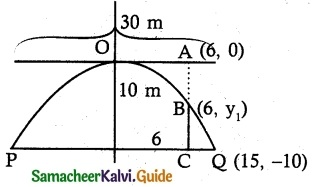PQ = 2a = 30 m
a = 15 m
Point Q be (15 , -10)
Equation of the parabola
x² = -4 ay …….. (1)
Q lies on parabola
15² = -4a( -10)
a = $$\frac {225}{40}$$
(1) ⇒ x² = -4($$\frac {225}{40}$$)y
x² = $$\frac {225}{10}$$y
Let B(6, y) lies on parabola
6² = $$\frac {225}{10}$$ y1
y1 = –$$\frac {36×10}{225}$$ = $$\frac {-8}{5}$$ = $$\frac {8}{5}$$ m
AB = AC – BC = 10 – $$\frac {8}{5}$$
= $$\frac {50-8}{5}$$ = $$\frac {42}{5}$$
AB = 8.4 m
∴ The height of the arch 6 m from the centre is 8.4 m.Question 2.
A tunnel through a mountain for a four lane highway is to have a elliptical opening. The total width of the highway (not the opening) is to be 16 m and the height at the edge of the road must be sufficient for a truck 4 m high to clear if the highest point of the opening is to be 5 m approximately. How wide must the opening be?
Solution: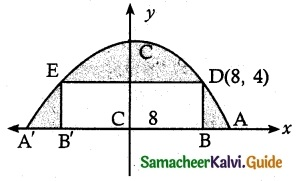Let the equation of the ellipse be
$$\frac {x^2}{a^2}$$ + $$\frac {y^2}{b^2}$$ = 1
Length of semi minor axis b = 5
i,e., $$\frac {x^2}{a^2}$$ + $$\frac {y^2}{5^2}$$ = 1
Let BB’ be the road width and AA’ be the end points of the opening of the tunnel.
Let CB = 8, BD = 4
∴ D is (8, 4) lies on the ellipse
$$\frac {8^2}{a^2}$$ + $$\frac {4^2}{5^2}$$ = 1
⇒ a² = $$\frac {25}{9}$$ × 64
⇒ a = $$\frac {40}{3}$$
The width AA’ = 2a
= $$\frac {80}{3}$$ = 26.66 m
The required width is 26.66 m.Question 3.
At a water fountain, water attains a maximum height of 4 m at horizontal distance of 0.5 m from its origin. If the path of water is a parabola, find the height of water at a horizontal distance of 0.75 m from the point of origin.
Solution: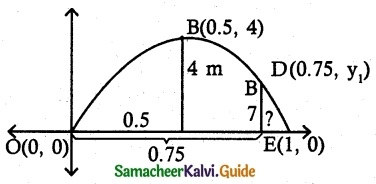Let the equation of parabola be
(x – h)² = -4a (y – k)
Here vertex B (0.5, 4)
∴ Equation of parabola
(x – 0.5)² = -4a(y – 4)
Parabola passes through origin (0, 0)
(0 – 0.5)² = -4a(0 – 4)
(-$$\frac {1}{2}$$)² = 16a
∴ $$\frac {1}{4}$$ = 16a ⇒ a = $$\frac {1}{64}$$
∴ Equation of parabola
(x – 0.5)² = -4($$\frac {1}{64}$$)(y- 4)
This Parabola passes again through D(0.75, y1)
∴ (0.75 – 0.5)² = –$$\frac {1}{16}$$ (y1 – 4)
(0.25)² = –$$\frac {1}{16}$$ (y1 – 4)
($$\frac {1}{4}$$)² = –$$\frac {1}{16}$$ (y1 – 4)
$$\frac {1}{16}$$ = –$$\frac {1}{16}$$ (y1 – 4)
1 = -y1 + 4
y1 = 3
Height of water at a horizontal distance of 0.75 m is 3 m.Question 4.
An engineer designs a satellite dish with a parabolic cross section. The dish is 5 m wide at the opening and the focus is placed 1.2 m from the vertex
(a) Position a co-ordinate system with the origin at the vertex and the x-axis on the parabola’s axis of symmetry and find an equation of the parabola.
(b) Find the depth of the satellite dish at the vertex.
Solution: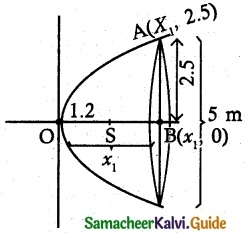(a) Consider the satellite dish is open rightward parabola
y2 = 4 ax ……….. (1)
Clearly a = 1.2m
(1) ⇒ y2 = 4(1.2)
y2 = 4.8x
(b) Use the point (x1, 2.5) in (1)
(2.5)2 = 4(1.2)x1
$$\frac{(2.5)^{2}}{4(1.2)}$$ = y1
x1 = 1.3 m
∴ The depth of the satellite dish at vertex is 1.3 mQuestion 5.
The parabolic cable of a 60 m portion of the roadbed of a suspension bridge are positioned as shown below. Vertical cables are to be spaced every 6 m along this portion of the roadbed. Calculate; the lengths of first two of these vertical cables: from the vertex.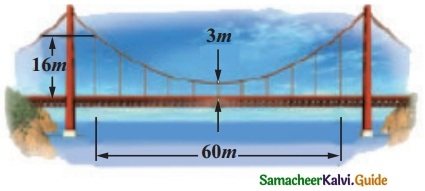Solution: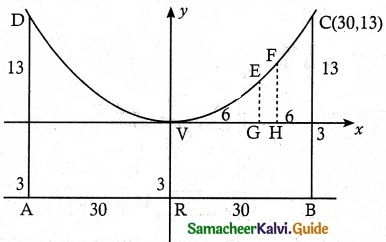From the diagram, the equation is x² = 4 ay and it passes through C(30, 13)
Equation of Parabola x² = 4ay.
30² = 4a × 13
4a = $$\frac {30^2}{13}$$
∴ Equation of the parabola is
x² = $$\frac {30^2}{13}$$y
(i) Let VG = 6 and GE = y
∴ E is (6, y) and it lies on the parabola
36 = $$\frac {30^2}{13}$$y
⇒ y = 0.52
Gable from the road = 3 + 0.52
= 3.52 m.

(ii) Let VH = 12 and HF = y
∴ F (12, y) lies on the parabola
12² = $$\frac {30^2}{13}$$y
⇒ y = $$\frac {144×13}{900}$$
= $$\frac {208}{100}$$y
y = 2.08
Cable from the road = 3 + 2.08 = 5.08
The heights of the first two vertical cables from the vertex are 3.52 m and 5.08 mQuestion 6.
Cross-section of a Nuclear cooling tower is in the shape of a hyperbola with equation $$\frac {x^2}{30^2}$$ – $$\frac {y^2}{44^2}$$ = 1. The tower is 150 m tall and the distance from the top of the tower to the centre of the hyperbola is half the distance from the base of the tower to the centre of the hyperbola. Find the diameter of the top and base of the tower.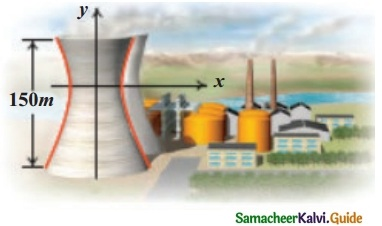Solution: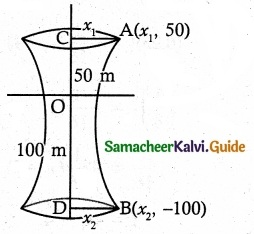Equation of hyperbola is $$\frac {x^2}{30^2}$$ – $$\frac {y^2}{44^2}$$ = 1
Given OC = $$\frac {1}{2}$$ OD and CD = 150
∴ OC = 50 m and OD = 100 m
Let the Radius of top of the tower be x1 and bottom of the tower be x2.
∴ Points A(x1, 50) and B(x2, 100)
Hyperbola passes through A(x1, 50)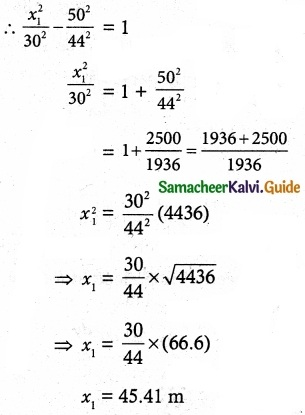∴ Radius of the top = 45.41 m.
Diameter of the top = 90.82 m
Also
The hyperbola again passes through B(x2, 100)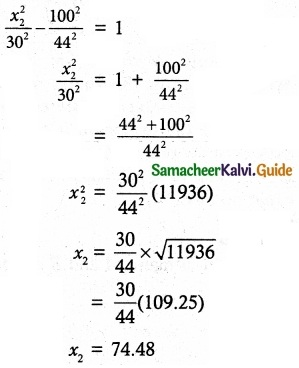∴ Radius of the base = 74.48 m.
Diameter of the base = 148.96 m
∴ Diameter of the top and base of the tower are 90.82 m and 148.96 m.Question 7.
A rod of length 1.2 m moves with its ends always touching the co-ordinate axes. The locus of a point P on the rod, which is
0. 3 m from the end in contact with x axis is an ellipse. Find the eccentricity.
Solution: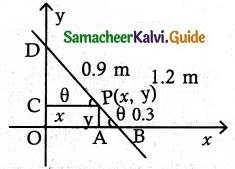Length of rod BD = 1.2 m
Let P(x, y) be any point on the Rod such
that PB = 0.3 m
∴ PD = 1.2 – 0.3 = 0.9 m
Let ΔPAB and ΔPCD are similar triangles
In ΔPAB sin θ = $$\frac {y}{0.3}$$
In A PCB cos θ = $$\frac {y}{0.9}$$
We know that sin² θ + cos² θ = 1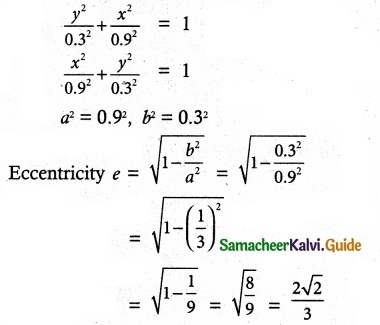Question 8.
Assume that water’issuing from the end of a horizontal pipe, 7.5 m. above the ground, describes a parabolic path. The vertex of the parabolic path is at The end of the pipe. At position 2.5 in below the line of the pipe, the flow of water has curved outward 3 m beyond the vertical, line through the end of the pipe. How far beyond this vertical line will the water strike the ground?
Solution: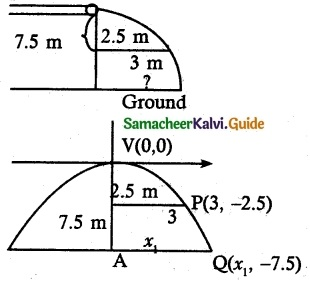Equation of the water path is
x2 = – 4 ay
Use the point (3, – 2.5) in (1)
(3)2 = – 4a(- 2.5)
9 = 10a
a = $$\frac{9}{10}$$ substituting in (1)
(1) ⇒ x2 = -4$$\frac{9}{10}$$y …………. (2)
Use the point (x1, -7.5) in (2)
(2) ⇒ x12 = -4 $$\frac{9}{10}$$(-7.5) ⇒ x12 = 30($$\frac{9}{10}$$)
x1 = $$\sqrt{3 \times 9}$$
x1 = $$3 \sqrt{3}$$ m
∴ The water strikes the ground $$3 \sqrt{3}$$ m beyond the vertical line.Question 9.
On lighting a rocket cracker it gets projected in a parabolic path and reaches a maximum height of 4 in when it is 6 m away from the point of projection. Finally, it reaches the ground 12 in away from the starting-point. Find the angle of! projection.
Solution: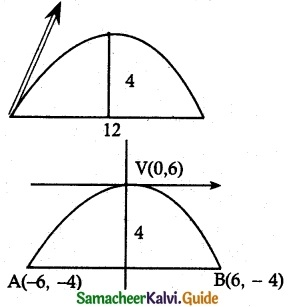Equation of the parabola be x² = – 4ay ……. (1)
B(6, -4) lies on parabola
6² = -4a(-4)
$$\frac {36}{16}$$ = a ⇒ a = $$\frac {9}{4}$$
(1) ⇒ x² = -($$\frac {9}{4}$$)y
x² = -9y ………. (2)
Now need tofind slope at (-6, -4)
Diff (2) w.r.to x
2x = -9 $$\frac {dy}{dx}$$
$$\frac {dy}{dx}$$ = $$\frac {2x}{-9}$$
At(-6, -4), $$\frac {dy}{dx}$$ = $$\frac {2(-6)}{-9}$$ = $$\frac {12}{9}$$ = $$\frac {4}{3}$$
tan θ = $$\frac {4}{3}$$
θ = tan-1($$\frac {4}{3}$$)Question 10.
Points A and B are 10 km apart and it is determined from the sound of an explosion heard at those points at different times that the location of the explosion is 6 km closer to A than 5. Show that the location of the explosion is restricted to a particular curve and find an equation of it.
Solution:
As shown in figure, A and B are on both sides of x-axis at Co-ordinates (-5, 0) and (5, 0)
The distance between A and B is 10. A point C is on the graph at Co-ordinates (x, y)
C is 6 km closer to A than B.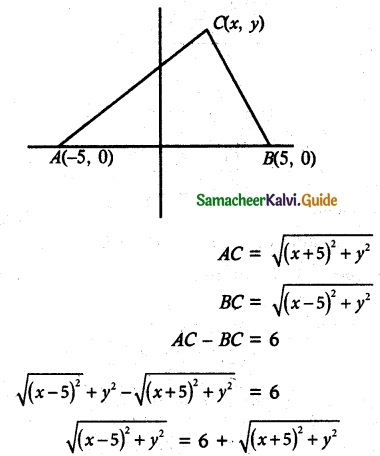Squaring on both sides we get,
(x – 5)² + y² = 36 + (x + 5)² + y² + 12($$\sqrt {(x+5)^2+y^2}$$)
x² + 25 – 10x + y² = x² + 10x + y² + 36 + 25 + 12$$\sqrt {(x+5)^2+y^2}$$
-20x – 36 = 12$$\sqrt {(x+5)^2+y^2}$$
(÷ by 4) ⇒ -5x – 9 = 3$$\sqrt {(x+5)^2+y^2}$$
Squaring both sides we get,
25x² + 81 + 90x = 9(x² + 25 + 10x + y²)
25x² + 81 + 90x – 9x² – 90x – 9y² – 225 = 0
16x² – 9y² – 144 = 0
16x² – 9y² = 144
(÷ by 144) ⇒ $$\frac {x^2}{9}$$ – $$\frac {y^2}{16}$$ = 1 is the required equation of hyperbola.